# 一、内容概要

• Evaluating a learning algorithm
• Deciding what to try next(决定接下来该试着做什么)
• Evaluating a Hypothesis (评估假设)
• Model Selection and Train/Validation/Test sets （模型选择和训练/验证/测试集划分）
• Bias vs. Variance
• Diagnosing(诊断) Bias vs. Variance
• Regularization and Bias/Variance
• Learning Curves(学习曲线)
• Deciding what to do next Revisited
• Buiding a Spam Classifier
• Priorizing what to work on
• Error Analysis
• Handling Skewed Data(处理偏斜数据)
• Error Metrics for Skewed Classes（不对称分类的错误评估）
• Trading Off Precision and Recall（精度与召回率的权衡）
• Using Large Data Sets
• Data For Machine Learning

# 二、重点&难点

## 1. Evaluating a Learning Algorithm

### 1） Deciding what to try next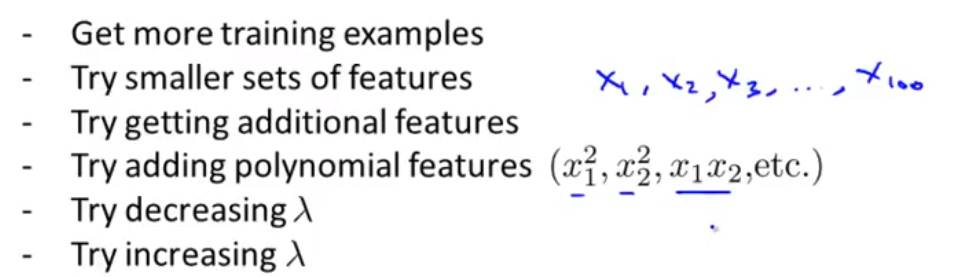### 2）Evaluating a Hypothesis

testError的计算方法依计算类型不同而不同：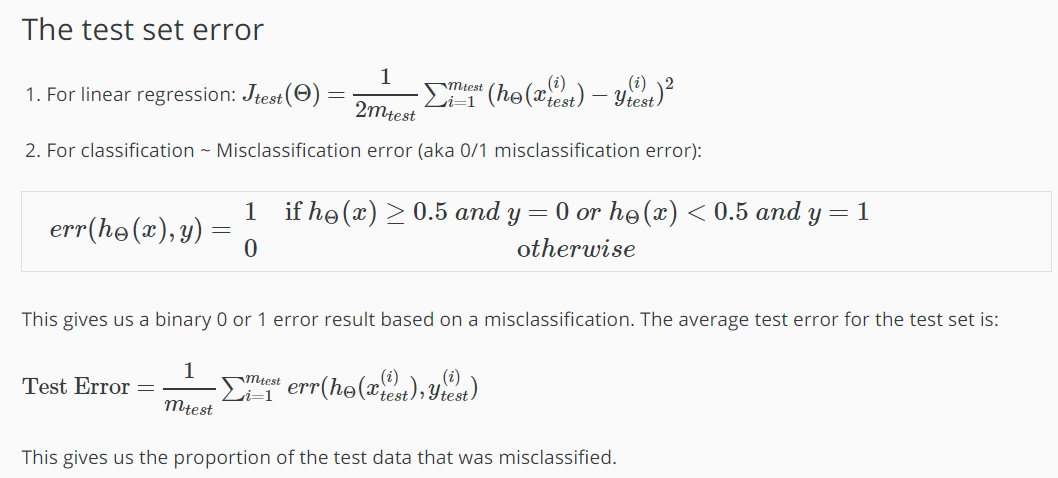### 3）Model Selection and Train/Validation/Test Sets

• Training Set（占60%左右）
• Cross validation Set（20%）
• Testing Set（20%）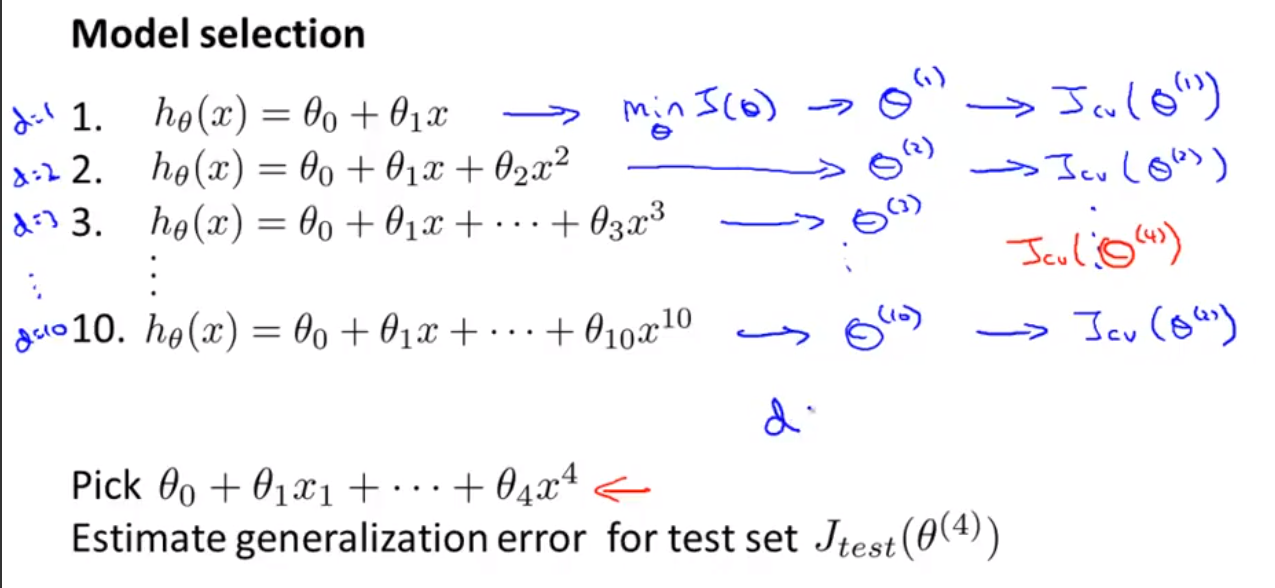1. 以Training Set为数据计算出10个不同的$$h_θ(x)$$θ参数
1. 将上一步中求出的θ参数分别代入验证数据集，并找出误差最小的一项，假设是$$θ^{(4)}$$
1. 最后将$$θ^{(4)}$$带入测试集，评估最后的整体误差

## 2. Bias vs. Variance

### 1) Diagnosing(诊断) Bias vs. Variance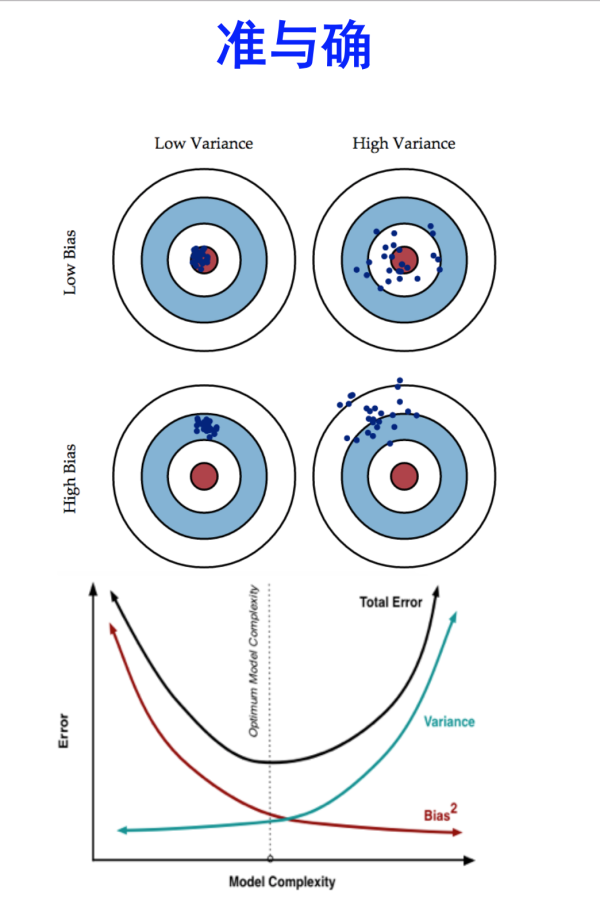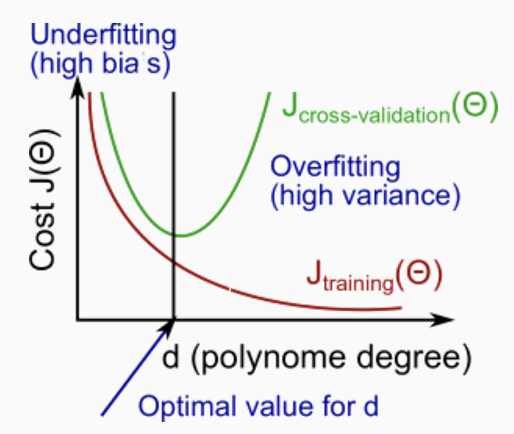### 2) Regularization and Bias/Variance

$h_θ(x) = θ_0 + θ_1x + θ_2x^2 +…… +θ_nx^n$
$J(θ) = \frac{1}{2m}\sum_{i=1}^{m}(h_θ(x^{(i)})-y^{(i)})^2+\frac{λ}{2m}\sum_{j=1}^{m}θ_j^2$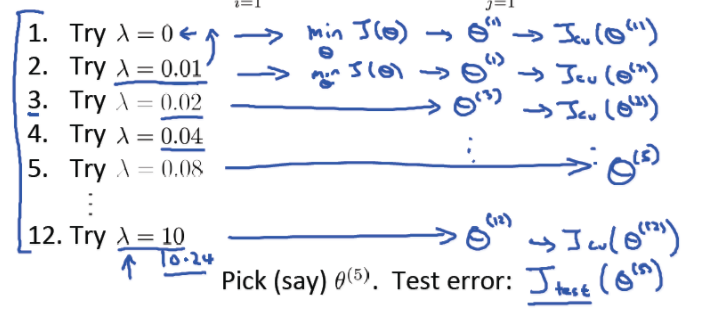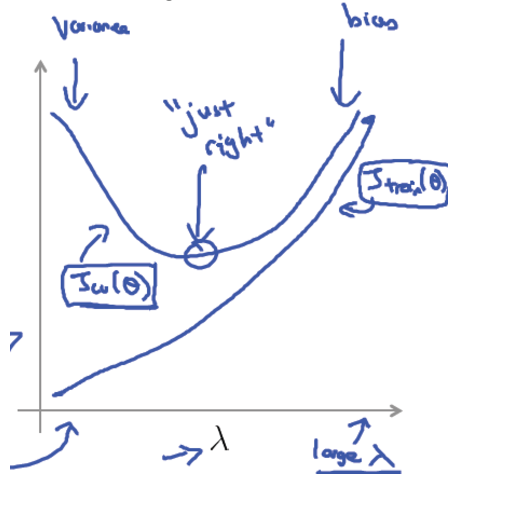• 1.创建一组λ的值，如λ∈{0,0.01,0.02,0.04,0.08,0.16,0.32,0.64,1.28,2.56,5.12,10.24});
• 2.创建一组不同degrees的$$h_θ(x)$$，即x的最高次幂不同
• 3.for λs in λ:
for hs in h:
学习得到一组θ
• 4.计算$$J_{CV}(θ)$$
• 5.选取验证集误差最小的一组参数
• 6.将上面求得的最佳θ和λ代入测试集求出$$J_{test}(θ)$$

### 3) Learning Curves

• 高偏差欠拟合问题的学习曲线：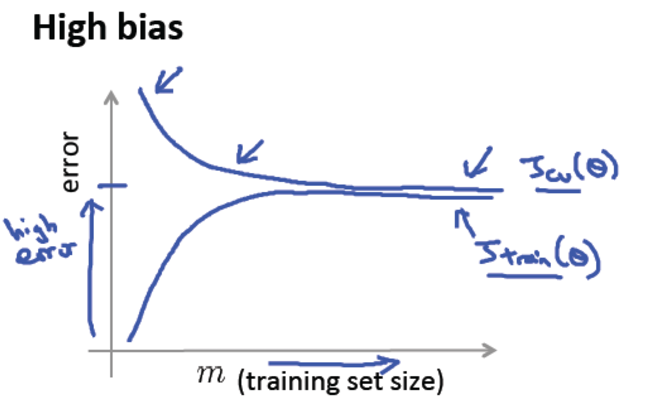• 高方差过拟合问题的学习曲线：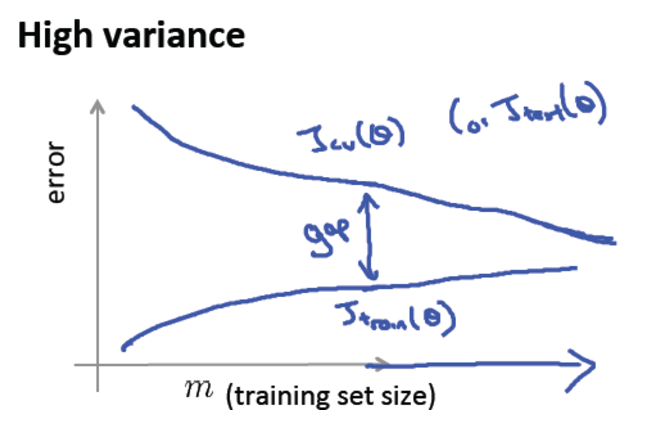### 4) Deciding what to do next Revisited

• 获取更多的训练样本

• 尝试使用更少的特征的集合

• 尝试获得其他特征

• 尝试添加多项组合特征

• 尝试减小 λ

• 尝试增加 λ

• 获取更多的训练样本 - 解决高方差

• 尝试使用更少的特征的集合 - 解决高方差

• 尝试获得其他特征 - 解决高偏差

• 尝试添加多项组合特征 - 解决高偏差

• 尝试减小 λ - 解决高偏差

• 尝试增加 λ -解决高方差

## 4.Handling Skewed Data

### 1) Error Metrics for Skewed Classes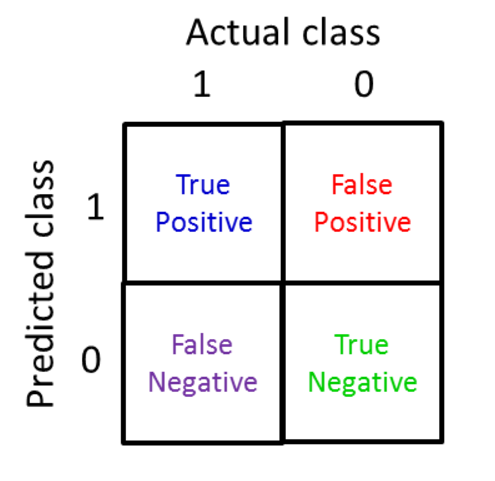True Positive （真正例, TP）被模型预测为正的正样本；可以称作判断为真的正确率

True Negative（真负例 , TN）被模型预测为负的负样本 ；可以称作判断为假的正确率

False Positive （假正例, FP）被模型预测为正的负样本；可以称作误报率

False Negative（假负例 , FN）被模型预测为负的正样本；可以称作漏报率

True/False: 指的是我们的算法预测分类的正负性
Postive/Negative: 指数据真实分类的正负性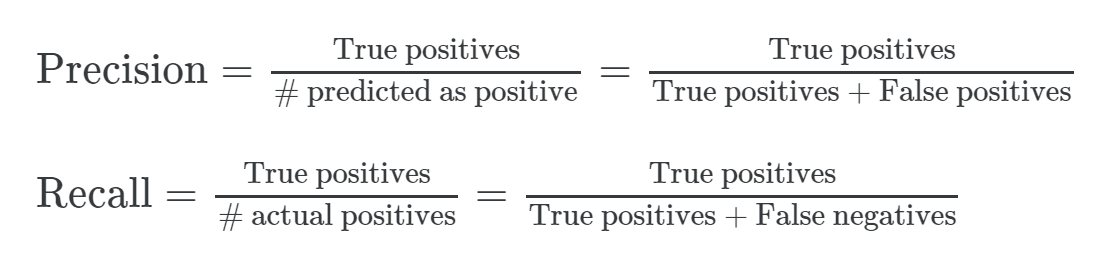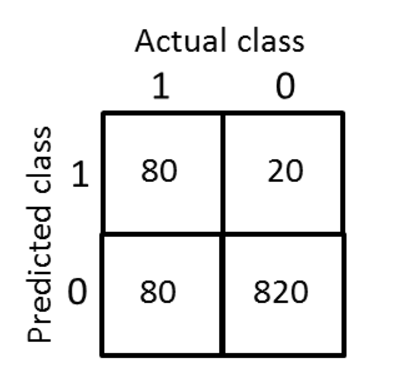$Precision = \frac{80}{80+20} = 0.8$
$Recall = \frac{80}{80+80} = 0.5$

Precision:预测中实际得癌症的病人数量(真正例)除以我们预测的得癌症的病人数量
Recall-预测中实际得癌症的病人数量(真正例)除以实际得癌症的病人数量

F值 = 70% * 50% * 2 / (70% + 50%) = 58.3%

F值 = 70% * 100% * 2 / (70% + 100%) = 82.35%

### 2) Trading Off Precision and Recall（精度与召回率的权衡）

• Precision1 = 0.85， Recall1 = 0.4
• Precision2 = 0.36， Recall2 = 0.90
这个时候再怎么评判呢？

• $$h_θ(x)≥0.5 ，则y=1$$
• $$h_θ(x)<0.5 ，则y=0$$
很显然我们知道阈值的大小的设定可以调节Precision和Recall，例如
• 当我们将阈值设为0.9，那么会导致高精度，低召回率（Higher precision, lower recall）
• 当我们将阈值设为0.3，那么会导致高召回率，低精确度(Higher recall, lower precision)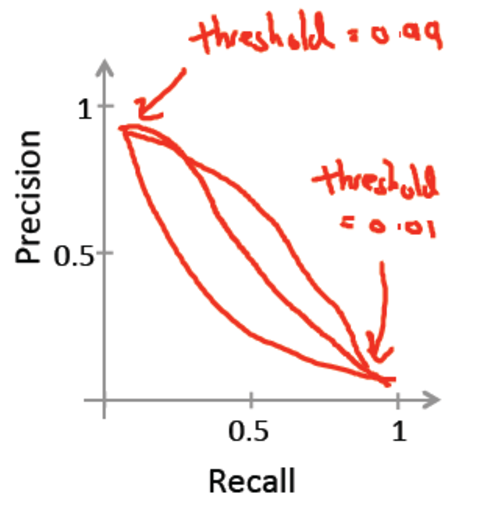$F1_{score} = 2\frac{P·R}{P+R}$

F值是对精确度和召回率的一个很好的权衡，两种极端的情况也能很好的平衡：

• P=0 R=0时，F1 = 0
• P=1 R=1时，F1 = 1

## 5.Using Large Data Sets

### MARSGGBO原创 2017-8-7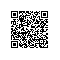使用钉钉扫一扫加入圈子
+ 订阅